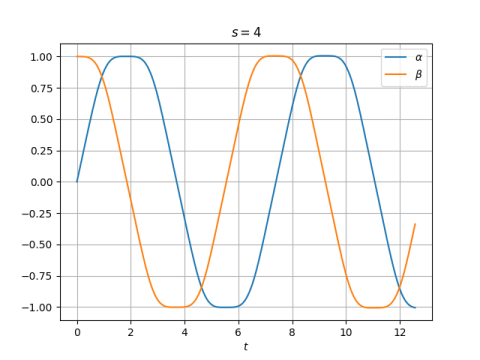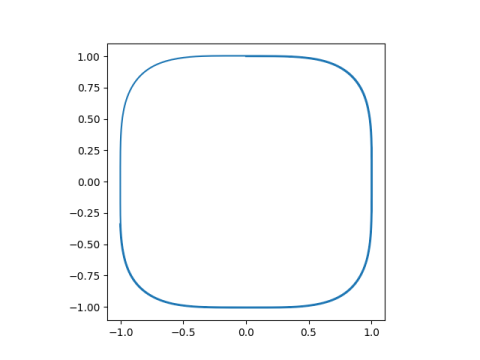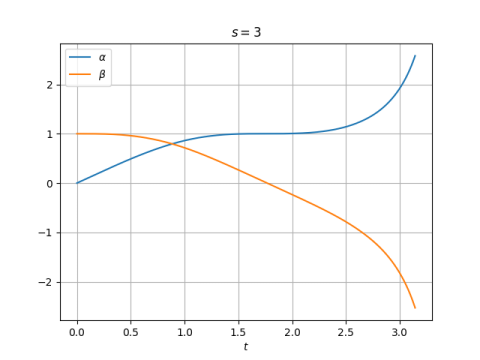# A generalization of sine and cosine

David Shelupsky  suggested a generalization of sine and cosine based on solutions to the system of differential equationswith initial conditions αs(0) = 0 and βs(0) = 1.

If s = 2, then α(t) = sin(t) and β(t) = cos(t). The differential equations above reduce to the familiar fact that the derivative of sine is cosine, and the derivative of cosine is negative sine.

For larger even values of s, the functions αs and βs look like sine and cosine respectively, though flatter at their maxima and minima. Numerical experiments suggest that the solutions are periodic and the period increases with s. 

Here’s a plot for s = 4.The first zero of α(t) is at 3.7066, greater than π. In the plot t ranges from 0 to 4π, but the second period isn’t finished.

If we look at the phase plot, i.e (α(t), β(t)), we get a shape that I’ve blogged about before: a squircle!This is because, as Shelupsky proved,## Odd order

The comments above mostly concern the case of even s. When s is odd, functions αs and βs don’t seem much like sine or cosine. Here are plots for s = 3and s = 5.## Other generalizations of sine and cosine

 David Shelupsky. A Generalization of the Trigonometric Functions. The American Mathematical Monthly, Dec. 1959, pp. 879-884

 After doing my numerical experiments I looked back more carefully at  and saw that the author proves that the solutions for even values of s are periodic, and that the periods increase with s, converging to 4 as s goes to infinity.

## 2 thoughts on “A generalization of sine and cosine”

1. Kaleberg

Do the curves for odd s look like chunky log and exp? (I wouldn’t be shocked.)

2. Michael

Thanks for the post! To get a sine/cosine-like solution for any real s >= 1, change the DEs to da/dt = sign(b) abs(b)^(s-1), db/dt = -sign(a) abs(a)^(s-1).

Also, I think the period goes from 4 to 8 as s goes from 1 to infinity (tested numerically). And the phase plot morphs from a diamond to a square.